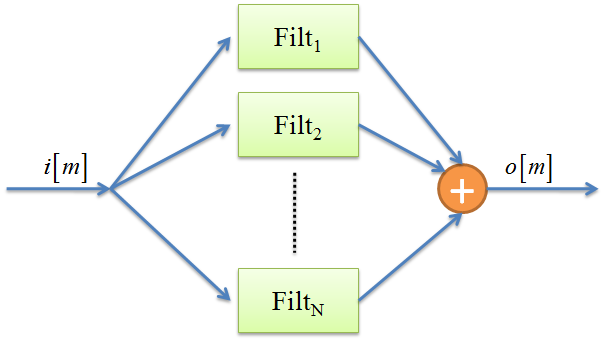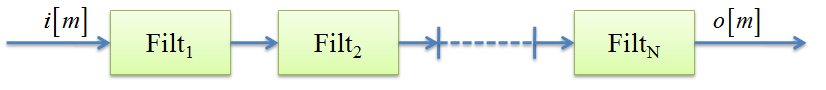# Filter banks

 Description Constructor description. Create Creating a filter bank in the LTPDA. From plist Creating from a plist. From filters Creating from filter objects. Import Importing an existing filterbank.

## Description

A filter bank is a container object for collections of MIIR and MFIR filters. The filter bank object can be used to filter a discrete data series in parallel or in series, in accordance to the schematic representations of figures 1 and 2.## Creating a filter bank in the LTPDA

The LTPDA Toolbox allows the implementation of filter banks by means of the filterbank class.

## Creating from a plist

The following example creates a parallel filter bank of MIIR filters.

```
iirhp = miir(plist('type', 'highpass'));
iirlp = miir(plist('type', 'lowpass'));
pl = plist('filters', [iirhp iirlp], ...
'type', 'parallel');
f = filterbank(pl)
```

The following example creates a serial filter bank of MIIR filters.

```
iirhp = miir(plist('type', 'highpass'));
iirlp = miir(plist('type', 'lowpass'));
pl = plist('filters', [iirhp iirlp], ...
'type', 'series');
f = filterbank(pl)
```

## Creating from filter objects

The following example creates a parallel filter bank of MIIR filters.

```
iirhp = miir(plist('type', 'highpass'));
iirlp = miir(plist('type', 'lowpass'));
pl = plist('type', 'parallel');
f = filterbank(iirhp,iirlp,pl)
```

The following example creates a serial filter bank of MIIR filters.

```
iirhp = miir(plist('type', 'highpass'));
iirlp = miir(plist('type', 'lowpass'));
pl = plist('type', 'series');
f = filterbank(iirhp,iirlp,pl)
```

## Importing an existing model

The filterbank constructor also accepts as an input existing models in different formats:

• XML files:

```    f = filterbank('foo_filterbank.xml')
```
• MAT files:

```    f = filterbank('foo_filterbank.mat')
```
• From repository:

```    f = filterbank(plist('hostname', 'localhost', 'database', 'ltpda', 'ID', []))
```